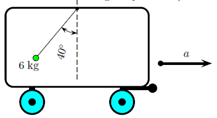Problem: A 6 kg object hangs at one end of a rope that is attached to a support on a railroad boxcar. When the car accelerates to the right, the rope makes an angle of 40◦ with the vertical The acceleration of gravity is 9.8 m/s2. Find the acceleration of the car. (Hint:  aobject = acar)

FREE Expert Solution
93% (500 ratings)
Problem Details

A 6 kg object hangs at one end of a rope that is attached to a support on a railroad boxcar. When the car accelerates to the right, the rope makes an angle of 40◦ with the vertical The acceleration of gravity is 9.8 m/s2. Find the acceleration of the car. (Hint:  aobject = acar)